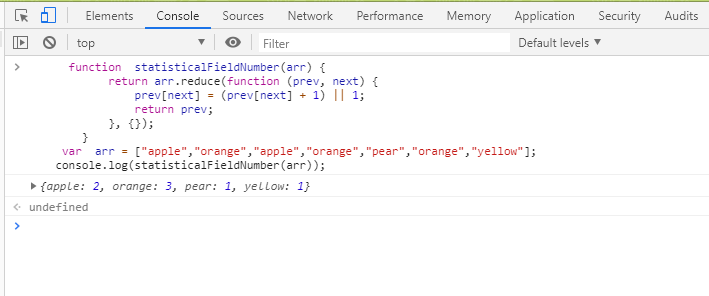• 今天小编就大家分享一篇用python一行代码得到数组中某个元素的个数方法，具有很好的参考价值，希望对大家有所帮助。一起跟随小编过来看看吧
• 使用numpy统计数组中0元素或图像中0像素值的个数，比直接遍历好很多。 import numpy as np a = np.array([[0,1,2],[2,0,0]]) cnt_array = np.where(a,0,1) print(np.sum(cnt_array)) 等于0的元素置1，求和即可。 ...
使用numpy自带的方法统计数组中0元素或图像中0像素值的个数，比直接遍历好很多。
import numpy as np
a = np.array([[0,1,2],[2,0,0]])
cnt_array = np.where(a,0,1)
print(np.sum(cnt_array))

等于0的元素置为1，求和即可。
展开全文python numpy
• 今天小编就大家分享一篇关于js根据需要计算数组中重复出现某个元素的个数，小编觉得内容挺不错的，现在分享给大家，具有很好的参考价值，需要的朋友一起跟随小编来看看吧
• 标题定义一个数组，查找数组中相同元素的个数 定义一个数组 int []arr={45,45,88,99,6,6,7,88}; 数组中有两个45，两个88，两个6，一个99，一个7. 代码如下 package Demo; public class Test { public static void...
标题定义一个数组，查找数组中相同元素的个数
定义一个数组 int []arr={45,45,88,99,6,6,7,88};数组中有两个45，两个88，两个6，一个99，一个7.代码如下
package Demo;
public class Test {
public static void main(String[] args) {
cc();
}
//查找数组中相同元素的个数
public static void cc() {
int []arr={45,45,88,99,6,6,7,88};
int []brr= new int[arr.length];
for (int i = 0; i < arr.length; i++) {
for (int j = 0,count=1; j < arr.length; j++) {
if(arr[i]==arr[j]){
brr[i]= count++;
}
}  System.out.println("brr[i] = " + brr[i]);
}

}
}

4输出结果如下： brr[i] = 2 brr[i] = 2 brr[i] = 1 brr[i] = 2 brr[i] = 2 brr[i] = 1 brr[i] = 2
展开全文java
• 今天去面试，面试官问我，给我一个数组数组中的数字都是不定大小的数字，问我怎么知道数组中0出现的个数。（例如：101算一个，100算两个）；当时我思考了一会，给出的答案是：遍历数组中的每一个值，传入一个递归...
今天去面试，面试官问我，给我一个数组，数组中的数字都是不定大小的数字，问我怎么知道数组中0出现的个数。（例如：101算一个，100算两个）；
当时我思考了一会，给出的答案是：遍历数组中的每一个值，传入一个递归的函数 getCount() 。函数先判断 num 是否为 0 ，是的话 n++ 并且 return 。否则计算其传入的值 num 对10取模后的大小 x ，然后判断 x 值是否为0，为0则n++,不为0则判断 num 是否小于10，是的话返回，不是的话则调用 getCount((num -x) /10) , 代码实现如下：
var arr = [10,20,1,11,101,324,561,89,210,1002,1030,1025,0];
var n = 0;
function getCount(num){
var x = num % 10;
if(num == 0){
n++;
return;
}
if(num < 10 && num != 0){
return;
}else if(x == 0){
n++;
getCount(num / 10);
}else{
getCount((num - x) / 10);
}
}
for(var i=0;i < arr.length;i++){
getCount(arr[i]);
}
然后面试管再跟我确认时，我感觉到好像有更好的实现方案，但是当时没想到，也就没有去更换答案了。
后面一想，我发现可以用另一种更加直白的方式实现。实现原理是基于JavaScript的字符串与数组间的转换，代码如下：
function getCount(arr){
var str = arr.join('');
var n = (str.split('0')).length-1;

}很简单就实现了这个功能。我真是想复杂了！


展开全文JavaScript
• (3)将二维数组转化一维 let arr = [ [ 0 , 1 ] , [ 2 , 3 ] , [ 4 , 5 ] ] let newArr = arr . reduce ( ( pre , cur ) => { return pre . concat ( cur ) } , [ ] ) console . log ...
      function  statisticalFieldNumber(arr) {
return arr.reduce(function (prev, next) {
prev[next] = (prev[next] + 1) || 1;
return prev;
}, {});
}
var  arr = ["apple","orange","apple","orange","pear","orange","yellow"];
console.log(statisticalFieldNumber(arr));

效果图：关于reduce的其他用法：
（1）数组求和，求乘积
var  arr = [1, 2, 3, 4];
var sum = arr.reduce((x,y)=>x+y)
var mul = arr.reduce((x,y)=>x*y)
console.log( sum ); //求和，10
console.log( mul ); //求乘积，24

(2)数组去重
let arr = [1,2,3,4,4,1]
let newArr = arr.reduce((pre,cur)=>{
if(!pre.includes(cur)){
return pre.concat(cur)
}else{
return pre
}
},[])
console.log(newArr);// [1, 2, 3, 4]

(3)将二维数组转化为一维
let arr = [[0, 1], [2, 3], [4, 5]]
let newArr = arr.reduce((pre,cur)=>{
return pre.concat(cur)
},[])
console.log(newArr); // [0, 1, 2, 3, 4, 5]

(4)将多维数组转化为一维
let arr = [[0, 1], [2, 3], [4,[5,6,7]]]
const newArr = function(arr){
return arr.reduce((pre,cur)=>pre.concat(Array.isArray(cur)?newArr(cur):cur),[])
}
console.log(newArr(arr)); //[0, 1, 2, 3, 4, 5, 6, 7]

(5)对象里的属性求和
var result = [
{
subject: 'math',
score: 10
},
{
subject: 'chinese',
score: 20
},
{
subject: 'english',
score: 30
}
];

var sum = result.reduce(function(prev, cur) {
return cur.score + prev;
}, 0);
console.log(sum) //60

展开全文• 问题：计算数组中不同元素的个数和 int len = ArrayNum; for (int i = 0; i &amp;amp;lt; ArrayNum; i++) { for (int j = i + 1; j &amp;amp;lt; ArrayNum; j++) { if (Array[j] == Array[i]) { // ...
• 如何计算数组中某项的个数<script type="text/javascript"> //统计一个数组中有多少个指定的数据项 var arr1= [12, 5, 8, 130, 44,5,51,5]; //方法一： function count(arr,num){ var total = 0; for (var i = ...filter find forEach
• public class Test { ... int[] oldArr = {1,3,4,5,6,6,0,5,6,7,0,5}; int[] copyNoZero = copyNoZero(oldArr); //调用Arrays工具类的方法，将数组转为字符串格式 String str = Arrays.toSt...javase
• 今天看到一道编程题：给定数组String[] a = {"a","b","c","d","a","b","a","c","e"}，查找数组中重复元素的个数：预期结果：a:3,b:2. /** * 查找数组里的重复元素个数 */ public static void ...
• #include<stdio.h> #include<iostream> #include<math.h> #include<algorithm> using namespace std; int a; int num={1};... int n,k=0,max=0; cin>...
• 问题描述 定义一个方法传入一个int类型数组，输出这个数组中每一个数字及其出现的个数 例如: 传入数组[1,2,2,2,3,3,4,4,4,4] 打印结果： 数字1出现了1次 数字2出现了3次… ...
• 现在我们定义了一个数组，如何获取数组中包含元素的个数呐？可以使用【sizeof(a)/sizeof(a)】求得。 sizeof()函数的作用是返回数组或某类型数据所占内存的字节个数。 所以使用sizeof(a)可以获得数组a占用的...笔记
• 设A[1..n]是包含n个不同数的数组，如果i而且A[i]>A[j]，则（i，j）一个逆序组，给出时间复杂度nlgn算法，确定n个任意元素排列逆序组的个数
• **字符数组中第一个元素非空字符。 #include<stdio.h> #define n 100 int main() { char a[n]; int i; int number=0; gets(a); //从键盘输入一段文字 if(a!='\0') { //该段文字不为空串 if(a...字符串 c语言
• 题意：一个整数数组，计算该数组中不相同整数的个数。 如A[]={1,8,2,6,7,8,5,5,4}则输出相同的个数为7. 方法一是使用hash数组，将hash数组里面的数全部制为0；然后hash[A[i]]为0时，将其设置1；最后hash数组的...字符串的转换 指针
• for(int i=0;i;i++){ num[arr[i]]++; } for(int j=0; j;j++) { if(num[j] != 0) { System.out.println(j+"出现了"+num[j]+"次"); } } } } 然后贴上运行结果 有什么疑问可以问我面试题 java
• // 返回数组中每个元素出现的次数 // 参数是任意数组,reduce的第2个参数要传一个空对象,这个空对象就是返回的个数的对象 var getNumCount = function(array) { return array.reduce((total,currentVal) => { if...
• import numpy as np import tensorflow as tf A = [1,3,4,5,6,1,2,3,4,5] B = [1,3,4,3,2,2,2,3,4,3] with tf.Session() as sess: a=sess.run(tf.equal(A, B)) b=a.tolist().count(True) print(a.tolist()) ...
• 假设有一个数组为：[1,2,3,4,3,2,2,6,3,1]，我们需要统计它里面每一个元素的个数 解题思路：对于这种统计类型的问题，我们可以创建一个空的对象。当一个对象没有指定的属性名时，会返回undefined。如下： let s ...es6 javascript
• 学python的第一天：计算数组中非重复元素的个数。 代码： -- coding: UTF-8 -- arr = [1,1,90,90,90,1000,2,1] brr = arr crr = arr n = len(arr) m = min(arr) i = 0 for j in range(0,n): crr = [] drr = [] for j...python
• 今天莫名的想到如果存在一个有元素重复出现的数组，那么如何求出这个数组中不同元素的个数。越想越不得解。然后搜索了关键词，居然找了大半天（百度的结果我都翻了好几页才找到满足要求的解答。）所以原创这篇博客，...
• python如何判断一个数组中有几个相同的数 输入 1 2 2 3 3 2 7 8 9 输出 6python
• 一、有时候的根据需要需要计算数组中某个元素重复出现的个数 var arr = ["apple","orange","apple","orange","pear","orange"]; 计算出来重复出现的...
•  * 用面向对象的方法求出数组中重复value的个数，  * 1 出现 1次  * 2出现 2次  *   * int arr[] = {1,4,65,2,4,6,2,3,4,4,1,5,1,3,4,98,1,1,96,2,1,9};  */     public static void main(String[] arg....
• 在笔试和面试经常会遇到这样的问题，统计字符串各个字符出现的个数， 对于统计频率一般通常的做法是利用map类数据结构，key作为原始元素的值，value作为频率 这里使用hashmap实现 import java.util.HashMap;...java hashmap 频次统计
• 逆序对： 在这样一个数组中，12和4就是一个逆序对，而15和85就不是...我们假设前面的数组为arr1，后面的数组为arr2，按照归并排序的思想，这两个数组已经是有序的了。 同时遍历两个数组，对于遍历到的两个数，比...
• 在JS，有时我们需要查询某个数组中指定元素的个数，并没有方法可以直接统计指定元素个数的，我们可以借助JS数组提供的filter()方法来间接实现。先来看看这个方法，代码如下： <script> var myArrays = new ...
• 本函数返回一个数组，其元素的键名是原数组的值，键值是该值在原数组中出现的次数。array_count_values(array)例如：&lt;?php\$a=array("Cat","Dog","Horse","Dog");...php...